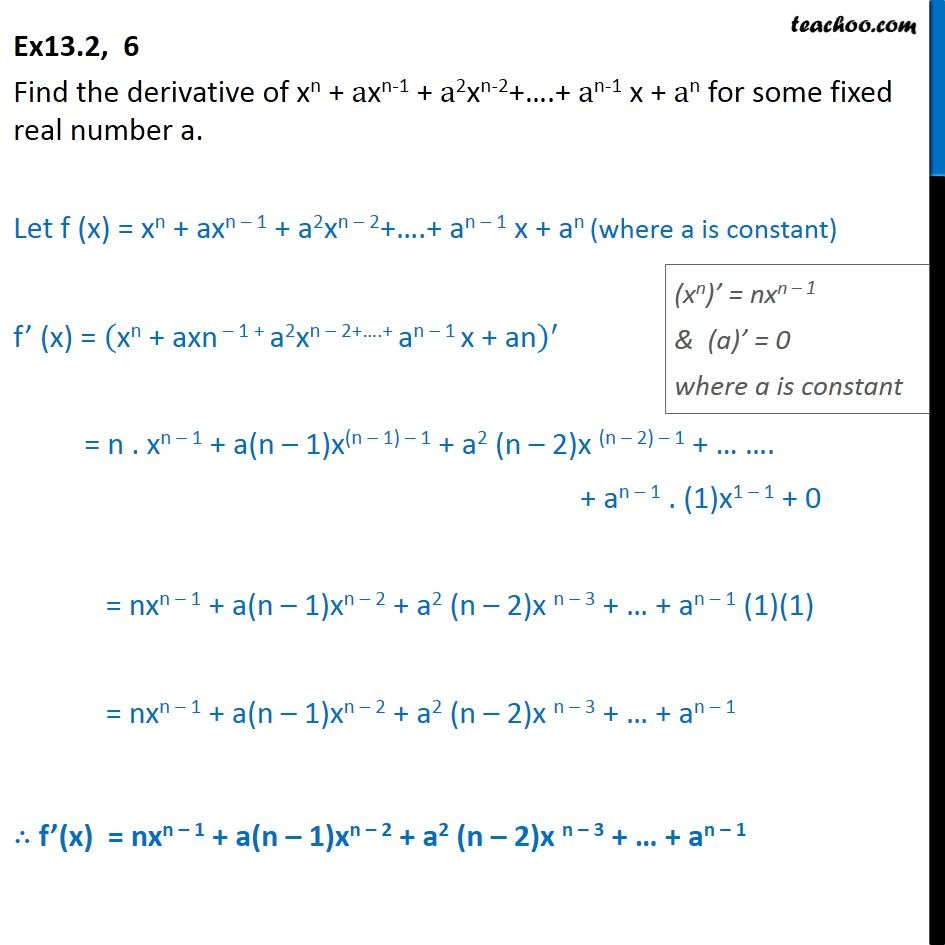1. Chapter 13 Class 11 Limits and Derivatives
2. Serial order wise
3. Ex 13.2

Transcript

Ex 13.2, 6 Find the derivative of xn + axn-1 + a2xn-2+….+ an-1 x + an for some fixed real number a. Let f (x) = xn + axn – 1 + a2xn – 2+….+ an – 1 x + an (where a is constant) f’ (x) = xn + axn – 1 + a2xn – 2+….+ an – 1 x + an﷯﷮′﷯ = n . xn – 1 + a(n – 1)x(n – 1) – 1 + a2 (n – 2)x (n – 2) – 1 + … …. + an – 1 . (1)x1 – 1 + 0 = nxn – 1 + a(n – 1)xn – 2 + a2 (n – 2)x n – 3 + … + an – 1 (1)(1) = nxn – 1 + a(n – 1)xn – 2 + a2 (n – 2)x n – 3 + … + an – 1 ∴ f’(x) = nxn – 1 + a(n – 1)xn – 2 + a2 (n – 2)x n – 3 + … + an – 1

Ex 13.2

About the AuthorDavneet Singh
Davneet Singh is a graduate from Indian Institute of Technology, Kanpur. He has been teaching from the past 10 years. He provides courses for Maths and Science at Teachoo.